# Support Vector Regression with R

In this article I will show how to use R to perform a Support Vector Regression.
We will first do a simple linear regression, then move to the Support Vector Regression so that you can see how the two behave with the same data.

## A simple data set

To begin with we will use this simple data set: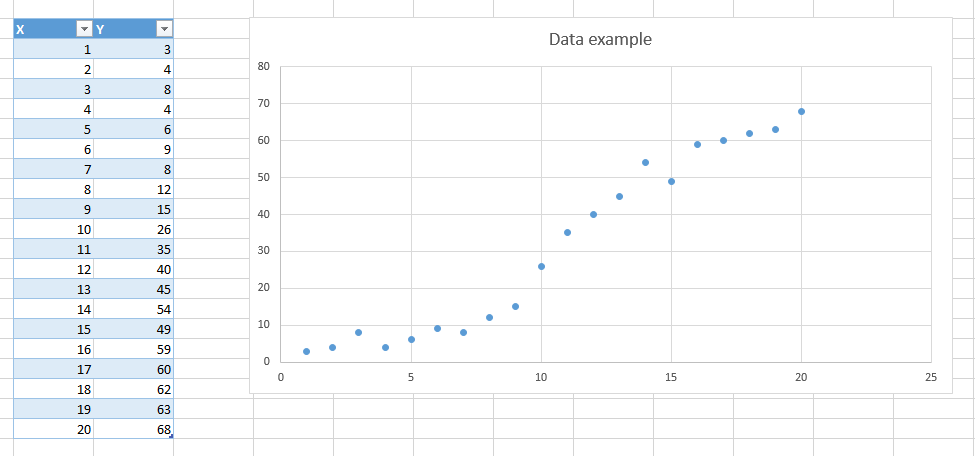I just put some data in excel. I prefer that over using an existing well-known data-set because the purpose of the article is not about the data, but more about the models we will use.

As you can see there seems to be some kind of relation between our two variables X and Y, and it look like we could fit a line which would pass near each point.

Let's do that in R !

## Step 1: Simple linear regression in R

Here is the same data in CSV format, I saved it in a file regression.csv :We can now use R to display the data and fit a line:

# Load the data from the csv file

# Plot the data
plot(data, pch=16)

# Create a linear regression model
model &lt;- lm(Y ~ X, data)

abline(model)


The code above displays the following graph:## Step 2: How good is our regression ?

In order to be able to compare the linear regression with the support vector regression we first need a way to measure how good it is.

To do that we will change a little bit our code to visualize each prediction made by our model

dataDirectory &lt;- "D:/"

plot(data, pch=16)
model &lt;- lm(Y ~ X , data)

# make a prediction for each X
predictedY &lt;- predict(model, data)

# display the predictions
points(data$X, predictedY, col = "blue", pch=4)  This produces the following graph: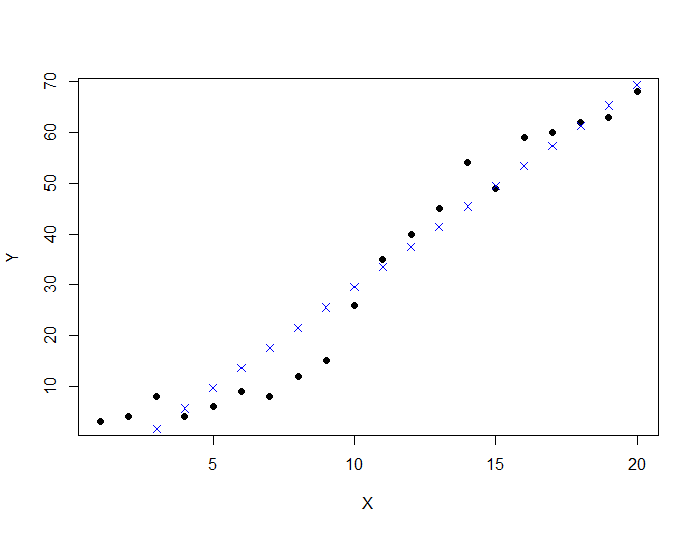For each data point $X_i$ the model makes a prediction $\hat{Y}_i$ displayed as a blue cross on the graph. The only difference with the previous graph is that the dots are not connected with each other. In order to measure how good our model is we will compute how much errors it makes. We can compare each $Y_i$ value with the associated predicted value $\hat{Y}_i$ and see how far away they are with a simple difference. Note that the expression $\hat{Y}_i - Y_i$ is the error, if we make a perfect prediction $\hat{Y}_i$ will be equal to $Y_i$ and the error will be zero. If we do this for each data point and sum the error we will have the sum of the errors, and if we takes the mean we will get the Mean Squared Error (MSE) A common way to measure error in machine learning is to use the Root Mean Squared Error (RMSE) so we will use it instead. To compute the RMSE we take the square root and we get the RMSE Using R we can come with the following code to compute the RMSE rmse &lt;- function(error) { sqrt(mean(error^2)) } error &lt;- model$residuals  # same as data$Y - predictedY predictionRMSE &lt;- rmse(error) # 5.703778  We know now that the RMSE of our linear regression model is 5.70. Let's try to improve it with SVR ! ## Step 3: Support Vector Regression In order to create a SVR model with R you will need the package e1071. So be sure to install it and to add the library(e1071) line at the start of your file. Below is the code to make predictions with Support Vector Regression: model &lt;- svm(Y ~ X , data) predictedY &lt;- predict(model, data) points(data$X, predictedY, col = "red", pch=4)



As you can see it looks a lot like the linear regression code. Note that we called the svm function (not svr !)  it's because this function can also be used to make classifications with Support Vector Machine. The function will automatically choose SVM if it detects that the data is categorical (if the variable is a factor in R).

The code draws the following graph: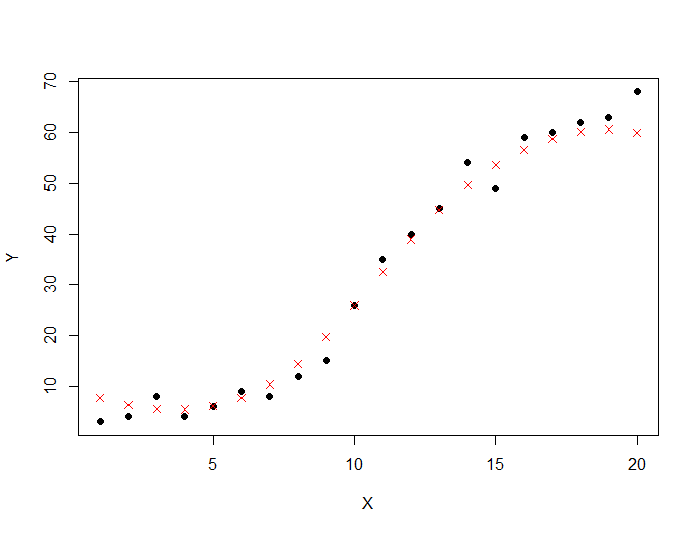This time the predictions is closer to the real values ! Let's compute the RMSE of our support vector regression model.

# /!\ this time  svrModel$residuals is not the same as data$Y - predictedY
# so we compute the error like this
error &lt;- data$Y - predictedY svrPredictionRMSE &lt;- rmse(error) # 3.157061  As expected the RMSE is better, it is now 3.15 compared to 5.70 before. But can we do better ? ## Step 4: Tuning your support vector regression model In order to improve the performance of the support vector regression we will need to select the best parameters for the model. In our previous example, we performed an epsilon-regression, we did not set any value for epsilon ( $\epsilon$ ), but it took a default value of 0.1. There is also a cost parameter which we can change to avoid overfitting. The process of choosing these parameters is called hyperparameter optimization, or model selection. The standard way of doing it is by doing a grid search. It means we will train a lot of models for the different couples of $\epsilon$ and cost, and choose the best one. # perform a grid search tuneResult &lt;- tune(svm, Y ~ X, data = data, ranges = list(epsilon = seq(0,1,0.1), cost = 2^(2:9)) ) print(tuneResult) # Draw the tuning graph plot(tuneResult)  There is two important points in the code above: • we use the tune method to train models with $\epsilon = 0, 0.1, 0.2, ... ,1$ and cost = $2^2, 2^3, 2^4, ... ,2^9$ which means it will train 88 models (it can take a long time) • the tuneResult returns the MSE, don't forget to convert it to RMSE before comparing the value to our previous model. The last line plot the result of the grid search: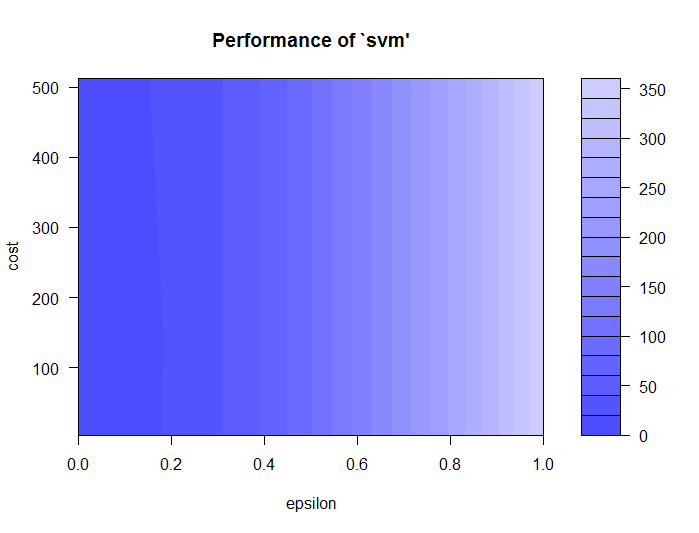On this graph we can see that the darker the region is the better our model is (because the RMSE is closer to zero in darker regions). This means we can try another grid search in a narrower range we will try with $\epsilon$ values between 0 and 0.2. It does not look like the cost value is having an effect for the moment so we will keep it as it is to see if it changes. tuneResult &lt;- tune(svm, Y ~ X, data = data, ranges = list(epsilon = seq(0,0.2,0.01), cost = 2^(2:9)) ) print(tuneResult) plot(tuneResult)  We trained different 168 models with this small piece of code. As we zoomed-in inside the dark region we can see that there is several darker patch. From the graph you can see that models with C between 200 and 300 and $\epsilon$ between 0.08 and 0.09 have less error.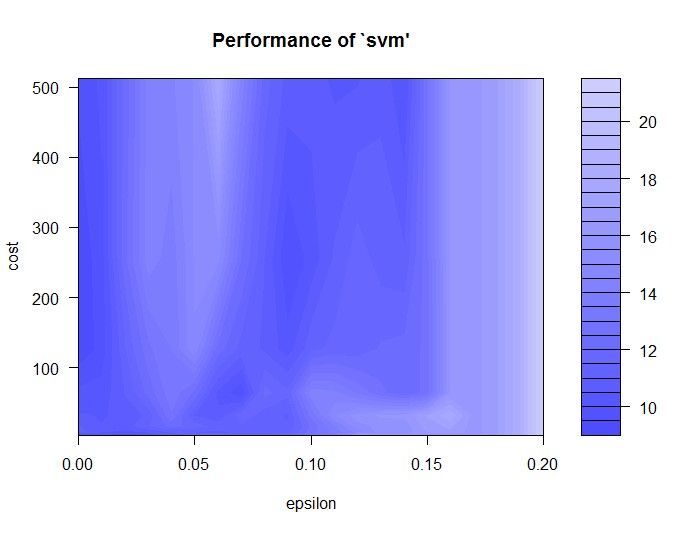Hopefully for us, we don't have to select the best model with our eyes and R allows us to get it very easily and use it to make predictions. tunedModel &lt;- tuneResult$best.model
tunedModelY &lt;- predict(tunedModel, data)

error &lt;- data$Y - tunedModelY # this value can be different on your computer # because the tune method randomly shuffles the data tunedModelRMSE &lt;- rmse(error) # 2.219642  We improved again the RMSE of our support vector regression model ! If we want we can visualize both our models. The first SVR model is in red, and the tuned SVR model is in blue on the graph below :I hope you enjoyed this introduction on Support Vector Regression with R. You can get the source code of this tutorial. Each step has its own file. If you want to learn more about Support Vector Machines, you can now read this article: An overview of Support Vector Machines ### 189 thoughts on “Support Vector Regression with R” 1.Hí, I have been studying and using the SVM. I work in a financial institution, and one of my responsabilities is to forecast the quarter budget for some variables, in this case non-performance-loans stock reduction. It happens that I trained a SVM model that fits my historic data very well. My question is : Ok you have this model, Then What ?! How could i use this trained model to predict future values ? ( I this case the next Quarter !) Reply •Your problem is not with SVM but with any machine learning model you could use. If your model fit your data and you make the assumption that it correctly represent and underlying unknown relation, then you input new data and use their result as prediction. Reply 2.Hi Alexandre, very nice explanation, thank you very much! Just a couple of questions (I am using a different dataset than yours): 1. when i predict the new data (my test set in my case) using the command: tunedModelY <- predict(tunedModel, data) --in my case data = test_set I get this error: "Error in scale.default(newdata[, object$scaled, drop = FALSE], center = object$x.scale$"scaled:center", :
length of 'center' must equal the number of columns of 'x'"
However, if I use the normal svm formula including the new tuned parameters manually, the command works (see code below):
tuned_regressor = svm(formula = Y ~ .,
data = training_set,
type = "eps-regression",
cost = 256,
epsilon = 0)
y_pred_best = predict(tuned_regressor, newdata = test_set)
Do you have any idea why?

2. Is it ever possible for the not tuned model to return a smaller RMSE than the tuned model? That's what happened in my case.

Thank you very much for taking the time to answer this.

Enrico

•Hello Enrico,
For 1. I can't give you help it really depends on your data.
For 2. Yes it is possible, that means that tuning did not improve the model. You can try to increase the range of the trained hyper parameters and see if it find a better model.

3.